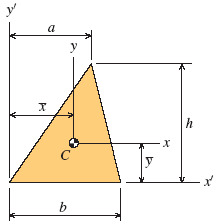Area Moment of Inertia Section Properties: Trapezoid Calculator

Section Properties Equations and Calculators

Area Moment of Inertia Section Properties: Oblique Trapezoid Section Properties Calculator

Area Moment of Inertia Section Properties of Oblique Trapezoid Calculator and Equations. This engineering calculator will determine the section modulus for the given cross-section. This engineering data is often used in the design of structural beams or structural flexural members.﻿
 Variables Inputs Inch mm Length h (in, mm) = Length b (in, mm) = Length a (in, mm) = Calculated Properties Area Moment of Inertia Section Ix (in4, mm4) = Area Moment of Inertia Section Ix' (in4, mm4) = Area Moment of Inertia Section Iy (in4, mm4) = Distance x (in, mm) = Distance y (in, mm) = Area (Unit2) =

Trapezoid Section Equation Used
 Description Equation Area Moment of Inertia Section Properties Ix (in4, mm4) = bh3 / 36 Area Moment of Inertia Section Properties Ix' (in4, mm4) = bh3 / 12 Area Moment of Inertia Section Properties Iy (in4, mm4) = (hb3 / 36) ( a2 - ab + b2 ) Distance to neutral axis y (in, mm) = h / 3 Distance to neutral axis x (in, mm) = ( a + b ) / 3 Area A (in2, mm2) = bh / 2Membership Register | LoginHomeEngineering Book StoreEngineering ForumExcel App. DownloadsOnline Books & ManualsEngineering NewsEngineering VideosEngineering CalculatorsEngineering ToolboxGD&T Training Geometric Dimensioning TolerancingDFM DFA TrainingTraining Online EngineeringAdvertising CenterCopyright Notice